Next: Spin Angular Momentum Up: Rydberg Formula Previous: Rydberg Formula

### Exercises

1. A particle of massis placed in a finite spherical well: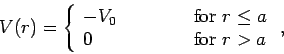withand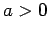. Find the ground-state by solving the radial equation with. Show that there is no ground-state if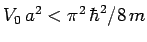.

2. Consider a particle of massin the three-dimensional harmonic oscillator potential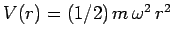. Solve the problem by separation of variables in spherical polar coordinates, and, hence, determine the energy eigenvalues of the system.

3. The normalized wavefunction for the ground-state of a hydrogen-like atom (neutral hydrogen,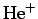,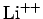, etc.) with nuclear chargehas the form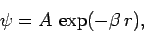whereand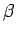are constants, andis the distance between the nucleus and the electron. Show the following:
1.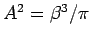.
2., where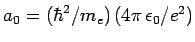.
3. The energy is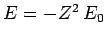where.
4. The expectation values of the potential and kinetic energies are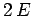and, respectively.
5. The expectation value ofis.
6. The most probable value ofis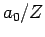.

4. An atom of tritium is in its ground-state. Suddenly the nucleus decays into a helium nucleus, via the emission of a fast electron which leaves the atom without perturbing the extranuclear electron, Find the probability that the resultingion will be left in an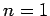,state. Find the probability that it will be left in a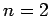,state. What is the probability that the ion will be left in an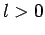state?

5. Calculate the wavelengths of the photons emitted from the,to,transition in hydrogen, deuterium, and positronium.

6. To conserve linear momentum, an atom emitting a photon must recoil, which means that not all of the energy made available in the downward jump goes to the photon. Find a hydrogen atom's recoil energy when it emits a photon in antotransition. What fraction of the transition energy is the recoil energy?Next: Spin Angular Momentum Up: Rydberg Formula Previous: Rydberg Formula
Richard Fitzpatrick 2010-07-20